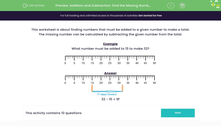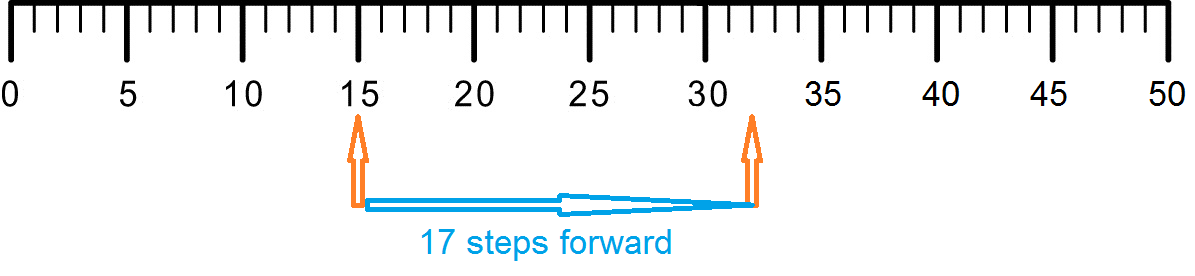# Choose Between Addition and Subtraction to Find a Missing Number

In this worksheet, students will explore the relationship between addition and subtraction to help them find missing numbers.Key stage:  KS 1

Curriculum topic:   Number: Addition and Subtraction

Curriculum subtopic:   Understand Relationship Between Addition and Subtraction

Difficulty level:#### Worksheet Overview

This activity is about finding numbers that must be added to a given number to make a total.

The missing number can be calculated in two different ways.

Example

What number must be added to 15 to make 32?

This means that we need to find the difference between 15 and 32.In the answer above, we have started at 15 and counted up to reach 32. This was 17 numbers forwards.

15 + 17 = 32

We can also find the difference between two numbers by using subtraction. If we had started at 32 on the number line, we could have counted back 15 numbers. We would have reached the number 17.

32 - 15 = 17

Both methods will give us the answer of 17. It is often the case that there is more than one way to solve a maths problem, so you need to decide which method works best for you or for the calculation that you are doing.

In this activity, you are going to be able to choose which method to use for each question. You may find that one method works better than another for different questions. Are you ready to have a go?### What is EdPlace?

We're your National Curriculum aligned online education content provider helping each child succeed in English, maths and science from year 1 to GCSE. With an EdPlace account you’ll be able to track and measure progress, helping each child achieve their best. We build confidence and attainment by personalising each child’s learning at a level that suits them.

Get started